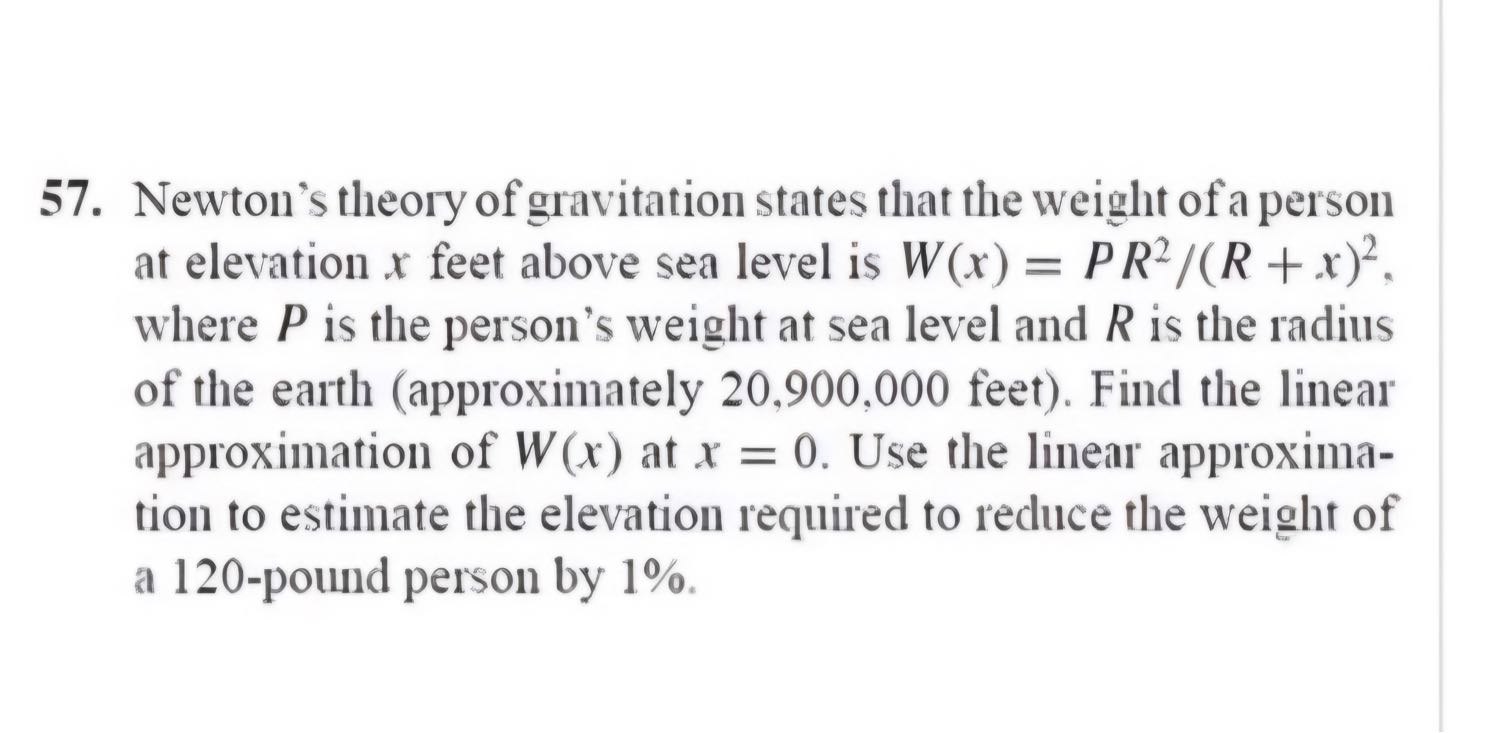﻿ โจทย์ Newton Method - Pantipติดตามพันทิป
ดาวน์โหลดได้แล้ววันนี้เกี่ยวกับเรา

## โจทย์ Newton Method

กระทู้คำถาม
โจทย์ข้อนี้ทำยังไงคะ ต้องทำโปรเจคเสนออาจารย์ แต่โจทย์ข้อนี้ งมมากก  รบกวนบอกบุญหน่อยจ้า 🙏🏻Newton's theory of gravitation states that the weight of a person at elevation x feet above sea level is W(x) = PR2/(R+x)². where P is the person's weight at sea level and R is the radius of the earth (approximately 20,900,000 feet). Find the linear approximation of W(x) at x = 0. Use the linear approxima- tion to estimate the elevation required to reduce the weight of a 120-pound person by 1%.
แสดงความคิดเห็น
โปรดศึกษาและยอมรับนโยบายข้อมูลส่วนบุคคลก่อนเริ่มใช้งาน อ่านเพิ่มเติมได้ที่นี่